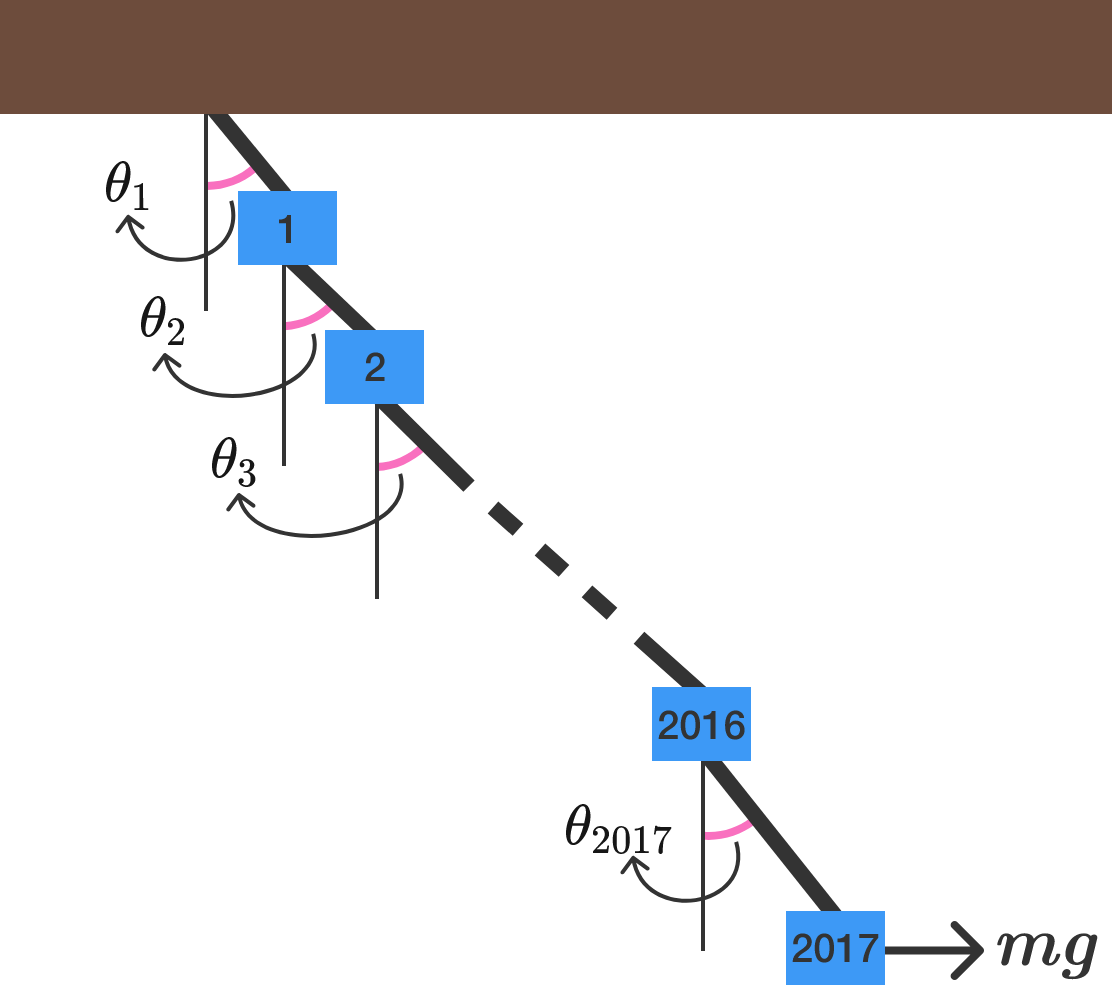# Problems of the Week

Contribute a problem

# 2017-04-03 Intermediate

$\large \underbrace{11111111\ldots 1}_{\text{77 one's}}$

Is this number prime or composite?Consider a cube with a thin rope attached to one of its vertices. The other endpoint of this rope is fixed to the bottom of a lightbulb which is hanging on the ceiling.

What is the shape of the shadow thrown on the floor by the cube?

Note: The only light source in the room is the lightbulb.

Bonus: If the light bulb is infinitely far away, what can we say about the shape?

Find the smallest positive integer $n$ such that

$\frac{n}{1},\ \ \frac{n-1}{2},\ \ \frac{n-2}{3},\ \ldots,\ \ \frac{n-2016}{2017}$ are all integers.

What are the last four digits of this number?While at the beach, Ted buried his friends in the sand with only their heads sticking out, as indicated in the image. Charles could see Ben and Andy only, Ben could see Andy only, and Andy and Donald couldn't see anyone.

Ted showed them 4 caps--2 black, 2 white. Ted then randomly placed these 4 caps on their heads, without showing them the color of their own cap. Without any communication, the 4 friends had to guess the color of the cap on their own head and whisper it into Ted's ear.

Find all of the people with the highest probability of guessing correctly.2017 blocks each of mass $m = \SI{20.17}{\kilogram}$ are connected by massless strings and the whole system is fixed to a rigid ceiling, as shown above.

A constant force $F = mg$ acts on the $2017^\text{th}$ block and displaces it. As a result, the entire system is disturbed and subsequently settles into a stationary equilibrium. In the equilibrium position, the string above block $i$ subtends an acute angle of $\theta_i$ with the vertical.

What is $\theta_1$ (in degrees) to 3 decimal places? Take acceleration due to gravity as $g=\SI[per-mode=symbol]{9.80}{\meter \per \second \squared}.$

×

Problem Loading...

Note Loading...

Set Loading...